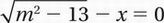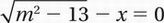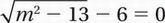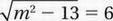# SAT Math Multiple Choice Question 652: Answer and Explanation

### Test Information

Question: 652

7.If m, 0 and x 5 6 in the equation above, what is the value of m?

• A. -13
• B. -10
• C. -7
• D. -3

Explanation:

C

Original equation:Substitute x = 6:Square both sides:

m2 - 13 = 36